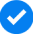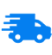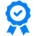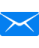•Lithium Specialists
•Free Shipping
•High-Quality Batteries
•Unrivaled Support

## The Beginner’s Guide to Current Handling in LiFePO4 Batteries

Posted on May 27 2020

## Current Handling in Lithium-ion Batteries

When it comes to deciding what to buy for your application, the most important, yet overlooked factor, is current handling.

Most people start the search for their lithium-Ion battery by looking at their voltage and capacity. Whilst considering the voltage is important, current handling is something that often gets forgotten about.

Furthermore, eBay product descriptions typically do not display everything you need to know about the products on offer. If they do, the information is usually confusing and unclear, leaving most people susceptible to a good bargain over quality, safety and performance.

That’s why Quality Source is here to give you the lowdown on just why it is important to know about current handling and how you can calculate your own needs. Not all lithium batteries are the same, so ensuring that you know about current handling in LiFePO4 batteries is critical to your safety, enjoyment and product longevity.

Before we dive into just what we should be looking out for when it comes to current handling in LiFePO4 batteries, let’s find out what current handling actually is.

Before you buy a new battery it is important to know how much energy you need to run your equipment.  There are two things you need to consider:

First of all, calculate the maximum amount of energy needed at any moment in time. To do this, simply add up all equipment that could be used at the same time.

This includes appliances like lights, fans, fridges etc. You’ll also need to take into account the amount of power that will be used by inverters - these typically use quite a lot of power, so ensure you get the continuous rate measurement for them.

Once you find the total amount of energy that all of your devices are going to use, you’ll know the maximum amount of power that is needed. From this, we can then calculate the continuous current handling that is needed. Typically this is to run laptop/ phone chargers, microwaves, induction hobs etc.  The total of all devices that could be used at once determines the maximum power that is needed, from this, we calculate the continuous current handling that is needed.

### How Much Power is Needed

The next step is to calculate how much power is used on average between charging with the wind, solar, motor etc, as well as how long they are used for. This is how we can work out how much battery capacity is needed.

We also need to understand how the watts of energy relates to amp-hours. 1 Watt is equivalent to one joule per second, which corresponds to the consumption rate of energy in an electric circuit. The potential difference of this is one volt and the current ampere-hour.

Simply put for DC 12V systems. Watts is a combination of Volts x Amps used:

VxA=W so 12Vx1A=12W.

We can work out current handling, by taking the maximum possible Watt usage, divided by  Voltage.

So if we add all the items which use energy at the same time, divided by the nominal Voltage, this equals the Amps needed to provide the energy required.

#### Example 1

If we had 5 LED lights which use 10W each, a Fridge which uses 100W, a water pump which uses 350W and a 1Kw Inverter to power a microwave, the total energy that can be used together is:

(5x10)=50+100+350+1000(1kW)=1500W.

We can then work out that:

1500/12V nominal voltage = 125 AMPS Current handling needed.

At full charge voltage 14V, the minimum amps needed is:

1500/14 = 107A

At the low state of charge 11V, the maximum amps needed is:

1500/11 = 136A

This shows that there can be a range of current handling needed depending on the state of charge.

#### Example 2

Using the same formula as above, however without the 1kW inverter, the power needed would be:

(5x10)=50+100+350= 500W

Current handling would be:

500/12 = 41.6A

At full charge 14V, the minimum current handling would be 35A.

At a low state of charge, it would be

650/11=45A

This is because inverters use lots of power. It is also important to understand that many inverters can have a 2kW or 3kW maximum energy drain.

So for a 12V nominal system, each kW of energy drain is approximately

(1kW)1000/12=83A

2kW=166A and 3kW=250Ah.

At a lower voltage from a low state of charge, this figure could be higher. At 11V this would be approximately 90A per kW.

At higher currents, the apparatus can become warmer, which can reduce voltage over longer wire lengths. This is why batteries are usually located close to charge sources and main current drain apparatus. At lower voltages, a much higher and thicker gauge wire is needed to be able to handle higher currents.

This is the reason as the demand for power on a boat or van goes above a certain level, 24V or 48V systems are used instead of 12V. If the same 1kW inverter is used in a 24V system, half the current handling is needed to do it compared to a 12V system.

1000/24=41.6A

Once we know the maximum current handling, it’s possible to decide whether we need a BMS with 100 Amp, 160 Amp or 200 Amp rating, And or whether we need more than one battery connected in Parallel to deliver what is required.

## How to Calculate Battery Storage Capacity

This section will aim to address the questions around how long we need our batteries to power our various energy needs before they need to be recharged again.

To work out the overall capacity required, we first need to estimate the average current drain, as well as how long this is needed in between recharges.

If 5 lights are used for 4 hours per day, a fridge compressor is used for say 12 hours per day and a water pump is used for 10 minutes per day:

Lights: 5 x 10W for 4 hours = 200Wh (Watts x Hours)

Fridge: 100W x 12hours = 1200Wh

Pump: 250W x 0.166 hours (10 minutes) = 41Wh.

Total per day = 1441Wh

Next, we take the total Wh used and divide by 12V:

1441/12=120Ah

In the above example, we used approximately 120Ah from a 12V battery per day. If we were to live ‘off-grid’ for 2 days with no solar, no engine and no mains charging, we would require a battery that can provide 120x2=240Ah of Usable energy.

We also need to decide how deep we want to discharge the battery. Our warranty is 2,000 cycles at 80% discharge, which is the most recommended discharge we would suggest.

If we were to take what we need in usable energy, then divide this by the decimal percentage (80% = 0.8):

240/0.8 = 300 Ah battery at 12V

In Example 1 above, we would suggest that to last 2 days, a battery of minimum capacity 300Ah is needed. This is sufficient enough to provide a regular 240Ah between charges, at an 80% discharge.

In this example, the maximum current handling needed is more than 100Amps and less than 160Aamps. We would recommend our Professional series 160A BMS battery. If this power was to be used for 2 days, then the 300Ah version would be appropriate.

Alternatively, our Novel Series 300Ah would last just as long yet provide even more spare capacity in current handling as the 200Ah and 300A  Novel series can also handle up to 200 Amps, through its WIFI enabled BMS.

It is good practice to ensure that any single battery can provide the current needs required in your system. So, for example, a boat could run on just 1 battery if required for short periods of time. This also avoids any issues with the potential for a cascade shut down. where there may be a temporary excess current drain. eg a motor starting for a winch, bow thruster or a big inverter being connected that can temporarily take a very big amount of power for a short time.

In the second example, the maximum current drain is under 100Ah, which means we can use our std HD 100A BMS, as this would meet the maximum current handling of 45Amps. A 300 Ah minimum HD Series battery is then required, to provide 2 full days running without any recharging.

We can increase the capacity above 300Ah total, which would drain the batteries to a lower SOC that will further extend the lifespan. If we were to install a total capacity of 480Ah, with the above example routinely using just 240Ah every 2 days before recharging, this would drain the batteries only 50%. This depth of discharge is associated with a lifespan of almost 6000 cycles, which is 3 times the guaranteed warranty cycle.

In addition to this, we could also run the system for just over 3 days (using 384Ah), with an 80% discharge. In practice, the customer needs to balance the cost of the batteries against their expected use and the number of cycles they would like the system to provide more than 80% of its original capacity.  2000 Cycles is the equivalent of 5 years of use discharging 1 x  per day.  6000 cycles would be about 15 years being completely drained once per day every day!!!  for most people any size using the example above between the recommended 300Ah minimum and 480Ah or more can be used. the less the bank is used the more cycles it will last for.

## Solar Charging

If solar panels were fitted, they help to feed the supply and partially charge the batteries in the daytime, these can reduce the amount of energy needed to be stored and may enable a smaller battery bank to be used.  However, it is worth considering that poor weather and unavoidable shadow on the panels can drastically reduce solar charging. For example, if we were situated in open land where there are fewer shady areas compared to woodland, there will be more access to solar gain as small areas of shadow on solar panels can greatly reduce their output.

## Final Thoughts

Quality Source is happy to help you choose the right system for your individual priorities and needs. Whether you have a low budget for Lithium systems or you require the ultimate performance with the latest most advanced batteries, don’t hesitate to get in touch today.